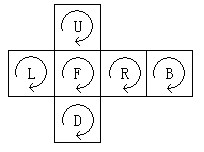# 2600. 2-Dimensional Rubik

Alex loves Rubik’s Cube. The 3-dimensional cubes are so difficult that Alex can only challenge the 2D ones, which have

six colors: W, Y, R, O, G, B.

Alex names the six faces as U(upper), D(bottom), L(left), R(right), F(front), B(back). The expanded cube will appear like

this:Alex is able to rotate any face 90 degrees in clockwise or counter-clockwise in a second. U, D, L, R, F, B are used for rotating the

corresponding face in clockwise, while U’, D’, L’, R’, F’, B’ are for counter-clockwise.

Now Alex got a Cube, he wants to know the minimum number of steps required for making one state to the other.

### 输入格式

The input consists of multiple test cases. The first line of input contains an integer T, which is the number of

test cases.

Each test case is on 12 lines. The first 6 lines indicate the initial state, while the next 6 lines indicate the destination state,

both according to the form of the sample input.

[Technical Specification]

T is an integer, and T <= 10.

You can assume that it is always possible to complete the task.

### 输出格式

For each test case, print the minimal steps required on a single line.

### 样例

Input
1
G W
Y G
W G O Y R B O R
Y B R W G B O B
W O
R Y
B B
B B
O O W W R R Y Y
O O W W R R Y Y
G G
G G

Output
10


2 人解决，5 人已尝试。

2 份提交通过，共有 9 份提交。

9.3 EMB 奖励。